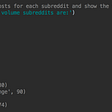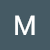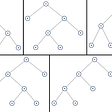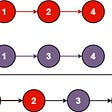# [Leet Code] Final Value of Variable After Performing Operations

`Input: operations = ["--X","X++","X++"]Output: 1Explanation: The operations are performed as follows:Initially, X = 0.--X: X is decremented by 1, X =  0 - 1 = -1.X++: X is incremented by 1, X = -1 + 1 =  0.X++: X is incremented by 1, X =  0 + 1 =  1.`
`Input: operations = ["++X","++X","X++"]Output: 3Explanation: The operations are performed as follows:Initially, X = 0.++X: X is incremented by 1, X = 0 + 1 = 1.++X: X is incremented by 1, X = 1 + 1 = 2.X++: X is incremented by 1, X = 2 + 1 = 3.`
`Input: operations = ["X++","++X","--X","X--"]Output: 0Explanation: The operations are performed as follows:Initially, X = 0.X++: X is incremented by 1, X = 0 + 1 = 1.++X: X is incremented by 1, X = 1 + 1 = 2.--X: X is decremented by 1, X = 2 - 1 = 1.X--: X is decremented by 1, X = 1 - 1 = 0.`
`class Solution(object):    def finalValueAfterOperations(self, operations):        """        :type operations: List[str]        :rtype: int        """                x = 0        for i in operations:            if "-" in i:                x -= 1            else:                x += 1        return x`

--

--

--

Learning, and posting my findings!

Love podcasts or audiobooks? Learn on the go with our new app.

## Top 10 FREE E-learning Authoring Tools for 2020## Lambda In Java## Introducing scalaps: Scala-inspired data structures for Python## Our favorite benchmarks for testing PCs and how to use them : Gadget Game News## Getting started with REST API Hygiene — I (Failure handling)## nextDAO Voting Result Announcement## Matthew Boyd

Learning, and posting my findings!

## All Possible Full Binary Trees Solution | LeetCode-894: Medium | JavaScript Implementation## Data Alignment in C++ : Part 1## What is a Queue?## LeetCode 21- Merge Two Sorted Lists